Next: Angle of Contact Up: Surface Tension Previous: Spherical Interfaces

# Capillary Length

Consider an interface separating the atmosphere from a liquid of uniform densitythat is at rest on the surface of the Earth. Neglecting the density of air compared to that of the liquid, the pressure in the atmosphere can be regarded as constant. On the other hand, the pressure in the liquid varies as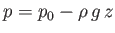(see Chapter 2), where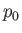is the pressure of the atmosphere,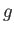the acceleration due to gravity, andmeasures vertical height (relative to the equilibrium height of the interface in the absence of surface tension). Note thatincreases upward. In this situation, the Young-Laplace equation, (3.12), yields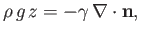(3.15)

where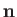is the normal to the interface directed from liquid to air. If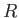represents the typical radius of curvature of the interface then the left-hand side of the previous equation dominates the right-hand side whenever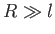, and vice versa. Here,(3.16)

is known as the capillary length, and takes the valuefor pure water at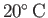(Batchelor 2000). We conclude that the effect of surface tension on the shape of an liquid/air interface is likely to dominate the effect of gravity when the interface's radius of curvature is much less than the capillary length, and vice versa.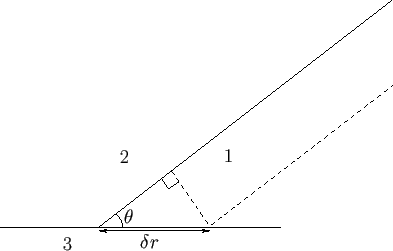Next: Angle of Contact Up: Surface Tension Previous: Spherical Interfaces
Richard Fitzpatrick 2016-03-31